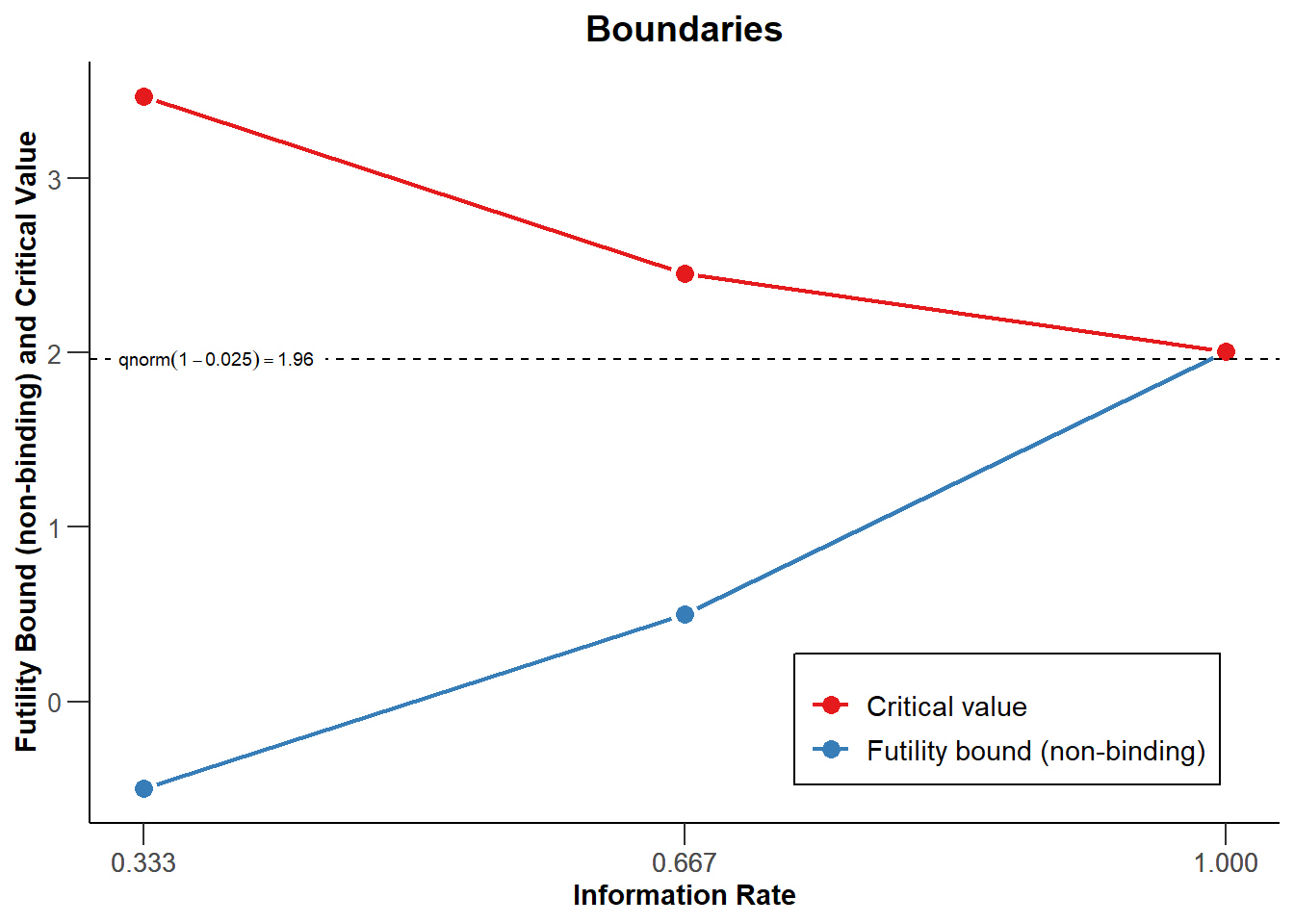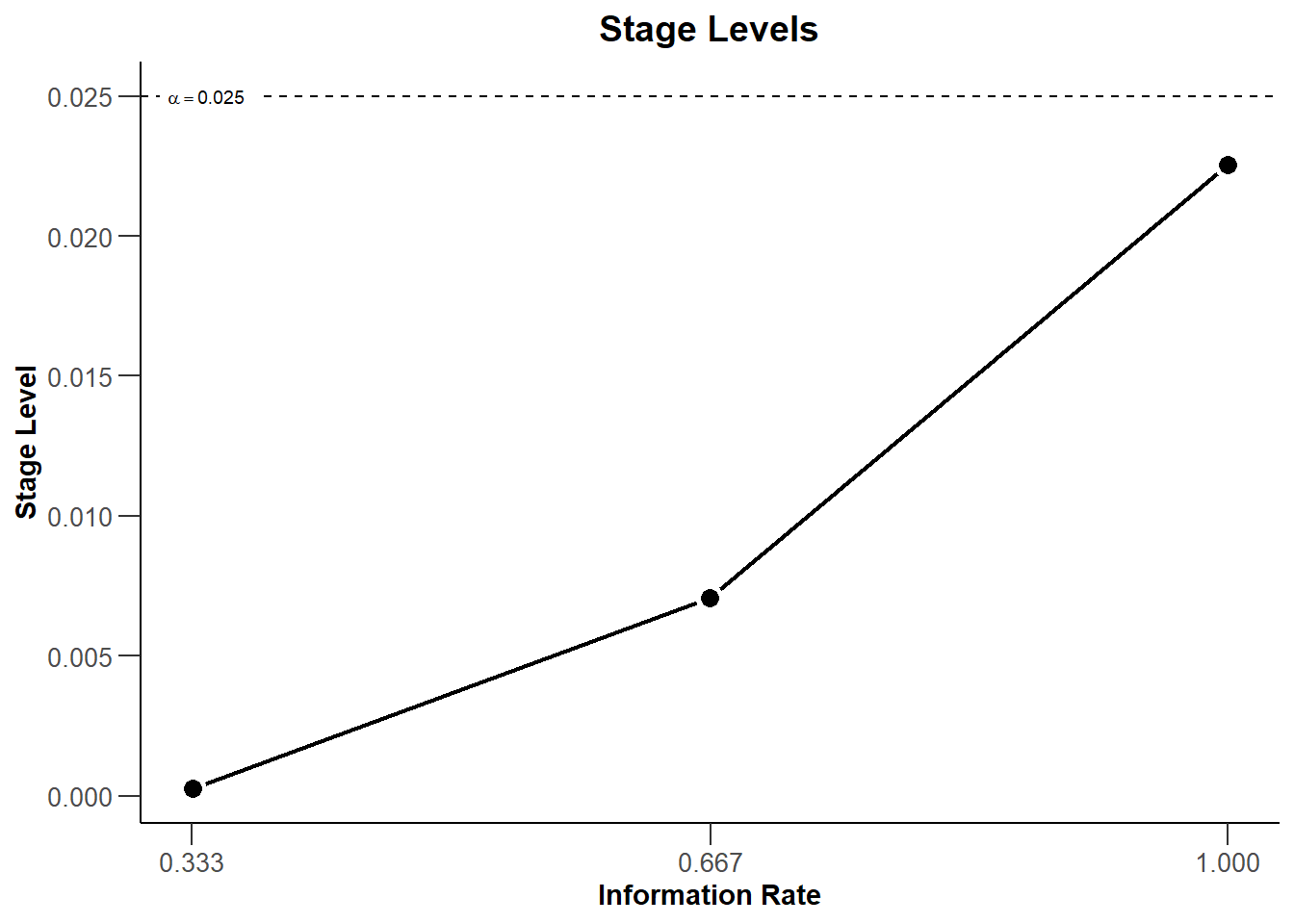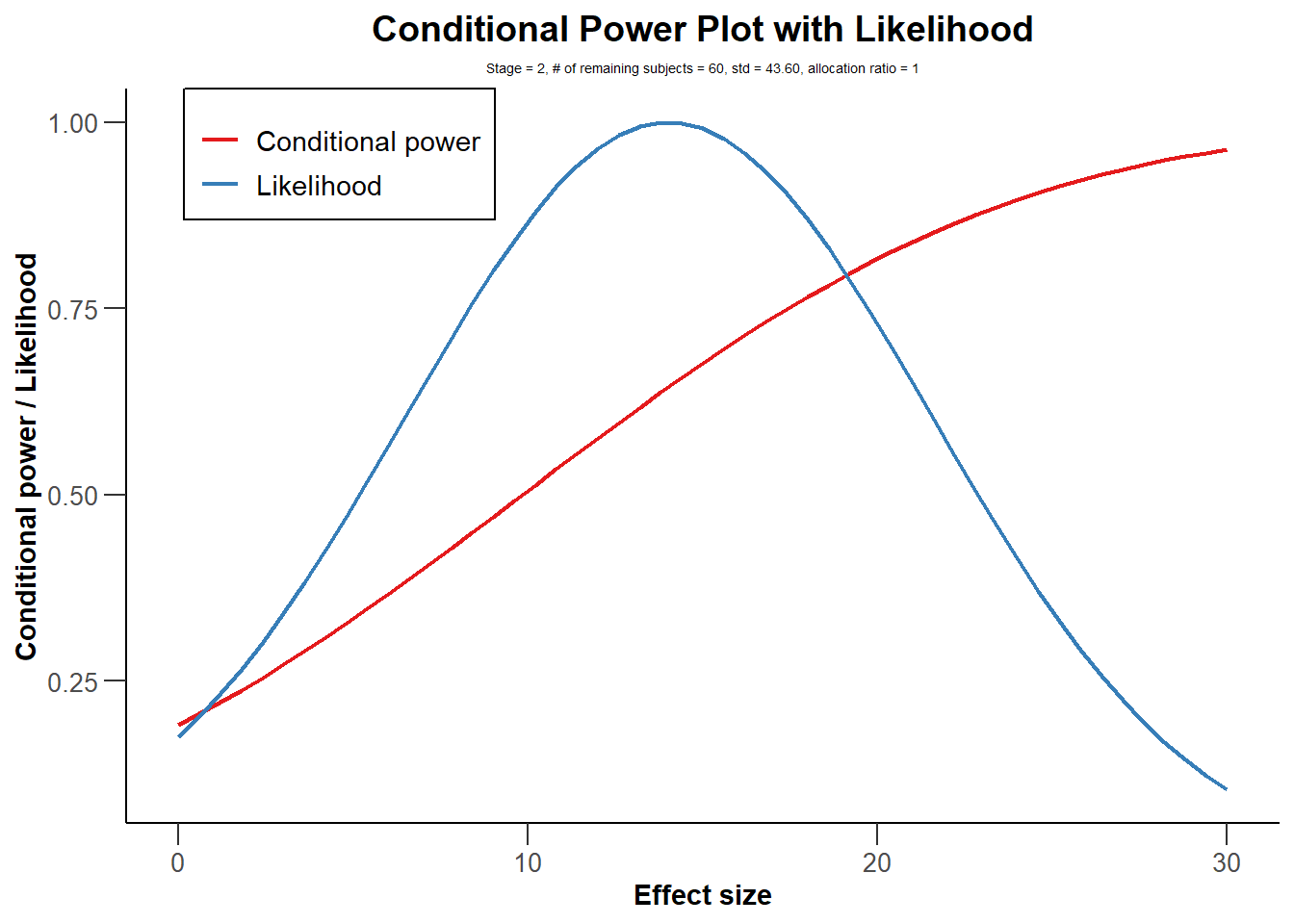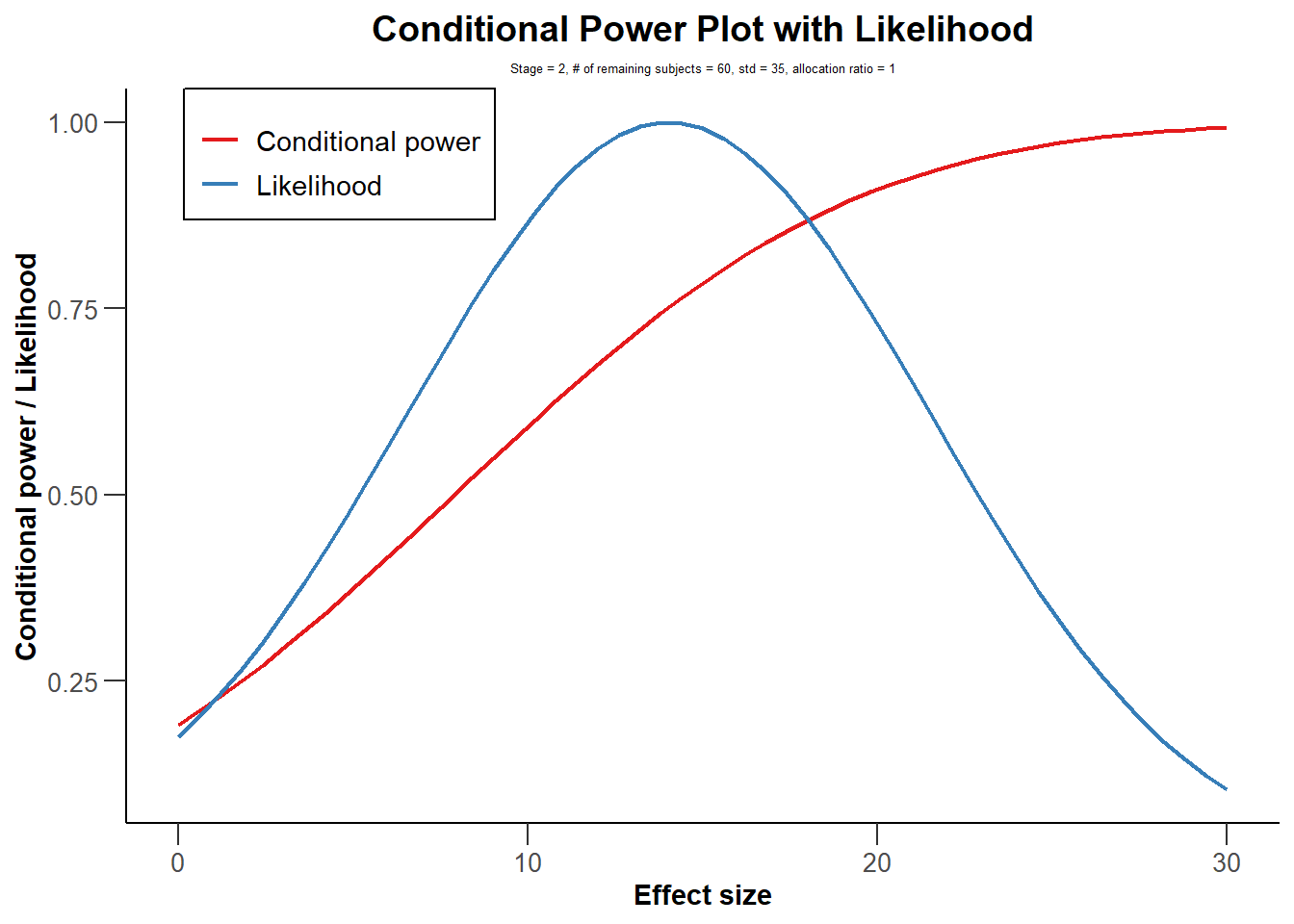# Summary

This R Markdown document provides an example for analysing trials with a continuous endpoint and sample size reassessment using rpact.

# 1 Define the type of design to be used for the analysis

library(rpact)
packageVersion("rpact") # version should be version 2.0.5 or later
##  '2.0.6'

Since we want to illustrate a design where at interim stages we are able to perform data-driven sample size adaptations, we use the inverse normal combination test for combining the $$p$$-values from the stages of the trial. We want to use a three stage design with OBrien and Fleming boundaries and additionally want to consider futility bounds -0.5 and 0.5 for the test statistics at the interim stages. Accordingly,

# Example of an inverse normal combination test:
designIN <- getDesignInverseNormal(futilityBounds = c(-0.5, 0.5))

defines the design to be used for this purpose. By default, this is a design with equally spaced information rates and one sided $$\alpha=0.025$$. The critical values can be displayed on the $$z$$-value or the $$p$$-value scale:

plot(designIN, type = 1)plot(designIN, type = 3)Note that we are using non-binding futility bounds that do not affect the boundaries for the rejection of the null hypothesis. It does have an effect, however, on the calculation of the conditional power.

By the use of the function getDesignInverseNormal() the way to analyse the data is fixed. With this definition, the unweighted inverse normal combination test is used, i.e., the stage results are combined through the use on the inverse normal combination test statistics

$\frac{\Phi^{-1}(1 - p_1) + \Phi^{-1}(1 - p_2)}{\sqrt{2}} \text{ and }\frac{\Phi^{-1}(1 - p_1) + \Phi^{-1}(1 - p_2)+ \Phi^{-1}(1 - p_3)}{\sqrt{3}}$

for the second and the third (final) stage of the trial, respectively.

# 2 Entering the data

In rpact, the way of using data for adaptive analysis is through summary statistics that summarize the data from the separate stages. Generally, the function getDataset() is used and depending on which summary statistics are entered, rpact knows the type of endpoint and the number of treatment groups. For testing means in a two-treatment parallel group design, means1, means2, stDevs1, stDevs2, n1, and n2 must be defined as vectors of length of the observed interim stages.

As an example, assume that the following results in the control and the experimental treatment arm were obtained for the first and the second stage of the trial.

First stage:

arm n mean std
experimental 34 112.3 44.4
control 37 98.1 46.7

Second stage:

arm n mean std
experimental 31 113.1 42.9
control 33 99.3 41.1

Here, sample size, mean and standard deviation were obtained separately from the stages. Enter these results as follows to obtain a dataset object in rpact:

dataSetExample <- getDataset(
means1  = c(112.3, 113.1),
means2  = c(98.1, 99.3),
stDevs1 = c(44.4, 42.9),
stDevs2 = c(46.7, 41.1),
n1      = c(34, 31),
n2      = c(37, 33))

The object

dataSetExample 

also contains the overall results which were calculated from the separate stage results:

## Dataset of means:
##   Stages                                       : 1, 1, 2, 2
##   Treatment groups                             : 1, 2, 1, 2
##   Sample sizes                                 : 34, 37, 31, 33
##   Means                                        : 112.3, 98.1, 113.1, 99.3
##   Standard deviations                          : 44.4, 46.7, 42.9, 41.1
##
## Calculated data:
##   Overall sample sizes                         : 34, 37, 65, 70
##   Overall means                                : 112.30, 98.10, 112.68, 98.67
##   Overall standard deviations                  : 44.40, 46.70, 43.35, 43.84

You might alternatively enter the overall results by specifying
overallMeans1, overallMeans2, overallStDevs1, overallStDevs2, overallN1, and overallN2:

getDataset(
overallMeans1  = c(112.3, 112.68),
overallMeans2  = c(98.1, 98.67),
overallStDevs1 = c(44.4, 43.35),
overallStDevs2 = c(46.7, 43.84),
overallN1      = c(34, 65),
overallN2      = c(37, 70))
## Dataset of means:
##   Stages                                       : 1, 1, 2, 2
##   Treatment groups                             : 1, 2, 1, 2
##   Overall sample sizes                         : 34, 37, 65, 70
##   Overall means                                : 112.30, 98.10, 112.68, 98.67
##   Overall standard deviations                  : 44.40, 46.70, 43.35, 43.84
##
## Calculated data:
##   Sample sizes                                 : 34, 37, 31, 33
##   Means                                        : 112.30, 98.10, 113.10, 99.31
##   Standard deviations                          : 44.40, 46.70, 42.90, 41.11

# 3 Analysis results

The easiest way to obtain the analysis results is through the function getAnalysisResults(), where design and dataInput needs to be specified. In our case,

getAnalysisResults(design = designIN, dataInput = dataSetExample, stage = 2)        

does the job, and the output is

## Analysis results (inverse normal design):
##   Stages                                       : 1, 2, 3
##   Information rates                            : 0.333, 0.667, 1.000
##   Critical values                              : 3.471, 2.454, 2.004
##   Futility bounds (non-binding)                : -0.500, 0.500
##   Cumulative alpha spending                    : 0.0002592, 0.0071601, 0.0250000
##   Stage levels                                 : 0.0002592, 0.0070554, 0.0225331
##   Effect sizes                                 : 14.20, 14.02
##   Test statistics                              : 1.310, 1.314, NA
##   p-values                                     : 0.09721, 0.09680, NA
##   Combination test statistics                  : 1.298, 1.837, NA
##   Actions                                      : continue, continue, NA
##   Theta H0                                     : 0
##   Cond. rejection probability                  : 0.06767, 0.19121, NA
##   Planned sample size                          : NA, NA, NA
##   Planned allocation ratio                     : 1
##   Assumed effect                               : 14.02
##   Assumed standard deviation                   : 43.6
##   Conditional power                            : NA, NA, NA
##   RCIs (lower)                                 : -25.271, -4.803, NA
##   RCIs (upper)                                 : 53.67, 32.80, NA
##   Repeated p-values                            : 0.29776, 0.07854, NA
##   Final stage                                  : NA
##   Final p-value                                : NA, NA, NA
##   Final CIs (lower)                            : NA, NA, NA
##   Final CIs (upper)                            : NA, NA, NA
##   Median unbiased estimate                     : NA, NA, NA

We note that the variable stage needs not to be specified because it is actually obtained from the data. You might, however, specify stage = 1 in order to obtain the results for the first stage only.

The inverse normal combination test statistic is calculated to be 1.837 which is smaller than 2.454. Hence, the hypothesis cannot be rejected, which is also reflected in the repeated $$p$$-value = 0.0785 larger than $$\alpha=0.025$$ and the lower bound of the repeated confidence interval = -4.803 being smaller than 0 (i.e., the RCI contains the null hypothesis value).

# 4 Reassessing the sample size for the last stage

The conditional power is calculated if nPlanned is specified. nPlanned contains the sample size of the remaining separate stages for both treatment groups and is a vector with that length. For example, if we specify nPlanned = 60, the conditional power is calculated for a total of 60 patients in the 2 treatment groups for the final stage with equal allocation between the treatment groups (the latter can be changed with allocationRatioPlanned which is 1 by default).

results <- getAnalysisResults(design = designIN, dataSetExample, stage = 2, nPlanned = 60)   

yields the following output:

## Analysis results (inverse normal design):
##   Stages                                       : 1, 2, 3
##   Information rates                            : 0.333, 0.667, 1.000
##   Critical values                              : 3.471, 2.454, 2.004
##   Futility bounds (non-binding)                : -0.500, 0.500
##   Cumulative alpha spending                    : 0.0002592, 0.0071601, 0.0250000
##   Stage levels                                 : 0.0002592, 0.0070554, 0.0225331
##   Effect sizes                                 : 14.20, 14.02
##   Test statistics                              : 1.310, 1.314, NA
##   p-values                                     : 0.09721, 0.09680, NA
##   Combination test statistics                  : 1.298, 1.837, NA
##   Actions                                      : continue, continue, NA
##   Theta H0                                     : 0
##   Cond. rejection probability                  : 0.06767, 0.19121, NA
##   Planned sample size                          : NA, NA, 60
##   Planned allocation ratio                     : 1
##   Assumed effect                               : 14.02
##   Assumed standard deviation                   : 43.6
##   Conditional power                            : NA, NA, 0.6449
##   RCIs (lower)                                 : -25.271, -4.803, NA
##   RCIs (upper)                                 : 53.67, 32.80, NA
##   Repeated p-values                            : 0.29776, 0.07854, NA
##   Final stage                                  : NA
##   Final p-value                                : NA, NA, NA
##   Final CIs (lower)                            : NA, NA, NA
##   Final CIs (upper)                            : NA, NA, NA
##   Median unbiased estimate                     : NA, NA, NA

The conditional power 0.645 is not very large and so a sample size increase might be appropriate. This conditional power calculation, however, is performed with the observed effect and observed standard deviation and so it might be reasonable to take a look at the effect size and its variability. This is graphically illustrated by the plot of the conditional power and the likelihood function over a range of alternative values. E.g., specify thetaRange = c(0,30) and obtain the graph below.

plot(results, thetaRange = c(0,30))You can also specify an alternative effect and standard deviation (e.g., thetaH1 = 15, assumedStDev = 35) for which the conditional power should be calculated, and graph the results. Here, the function getConditionalPower() together with getStageResults() are used but you obtain the same result when specifying thetaH1 = 15, assumedStDev = 35 in getAnalysisResults().

stageResults <- getStageResults(design = designIN, dataInput = dataSetExample)

getConditionalPower(design = designIN, stageResults, nPlanned = 60, thetaH1 = 15,
assumedStDev = 35)
## $nPlanned ##  NA NA 60 ## ##$conditionalPower
##         NA        NA 0.7841792
plot(stageResults, nPlanned = 60, thetaRange = c(0,30), assumedStDev = 35)# 5 Final analysis

Assume now that it was decided to continue with the originally planned sample size (= 60 per stage) and the final stage shows the results:

Final stage:

arm n mean std
experimental 32 111.3 41.4
control 31 100.1 39.5

We obtain the test results for the final stage as follows:

dataSetExample <- getDataset(
means1  = c(112.3, 113.1, 111.3),
means2  = c(98.1, 99.3, 100.1),
stDevs1 = c(44.4, 42.9, 41.4),
stDevs2 = c(46.7, 41.1, 39.5),
n1      = c(34, 31, 32),
n2      = c(37, 33, 31))

getAnalysisResults(design = designIN, dataInput = dataSetExample)    
## [PROGRESS] Stage results calculated [0.015 secs]
## [PROGRESS] Conditional power calculated [0 secs]
## [PROGRESS] Conditional rejection probabilities (CRP) calculated [0 secs]
## [PROGRESS] Repeated confidence interval of stage 1 calculated [0.4484 secs]
## [PROGRESS] Repeated confidence interval of stage 2 calculated [0.4189 secs]
## [PROGRESS] Repeated confidence interval of stage 3 calculated [0.3793 secs]
## [PROGRESS] Repeated confidence interval calculated [1.2477 secs]
## [PROGRESS] Overall repeated p-values of stage 1 calculated [0.2192 secs]
## [PROGRESS] Overall repeated p-values of stage 2 calculated [0.2332 secs]
## [PROGRESS] Overall repeated p-values of stage 3 calculated [0.2422 secs]
## [PROGRESS] Repeated p-values calculated [0.6947 secs]
## [PROGRESS] Final p-value calculated [0.001 secs]
## Warning: Calculation of final confidence interval performed for kMax = 3 (for
## kMax > 2, it is theoretically shown that it is valid only if no sample size
## change was performed)
## [PROGRESS] Final confidence interval calculated [0.0591 secs]
## Analysis results (inverse normal design):
##   Stages                                       : 1, 2, 3
##   Information rates                            : 0.333, 0.667, 1.000
##   Critical values                              : 3.471, 2.454, 2.004
##   Futility bounds (non-binding)                : -0.500, 0.500
##   Cumulative alpha spending                    : 0.0002592, 0.0071601, 0.0250000
##   Stage levels                                 : 0.0002592, 0.0070554, 0.0225331
##   Effect sizes                                 : 14.20, 14.02, 13.12
##   Test statistics                              : 1.310, 1.314, 1.098
##   p-values                                     : 0.09721, 0.09680, 0.13826
##   Combination test statistics                  : 1.298, 1.837, 2.128
##   Actions                                      : continue, continue, reject
##   Theta H0                                     : 0
##   Cond. rejection probability                  : 0.06767, 0.19121, NA
##   Planned sample size                          : NA, NA, NA
##   Planned allocation ratio                     : 1
##   Assumed effect                               : 13.12
##   Assumed standard deviation                   : 42.43
##   Conditional power                            : NA, NA, NA
##   RCIs (lower)                                 : -25.2714, -4.8030, 0.7676
##   RCIs (upper)                                 : 53.67, 32.80, 25.31
##   Repeated p-values                            : 0.29776, 0.07854, 0.01828
##   Final stage                                  : 3
##   Final p-value                                : NA, NA, 0.01968
##   Final CIs (lower)                            : NA, NA, 0.6209
##   Final CIs (upper)                            : NA, NA, 24.52
##   Median unbiased estimate                     : NA, NA, 12.62`

The warning indicates that the calculation of the final CI can be critical if sample size changes were performed. This is not the case here, and so the confidence interval that is based on the stagewise ordering given by (0.62; 24.52) is a valid inference tool. Together with the RCI at stage 3 (0.77; 25.31) it corresponds to the test decision of rejecting the null hypothesis. The test decision is also reflected in both the final $$p$$-value and repeated $$p$$-value being smaller than $$\alpha=0.025$$.